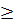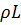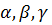### EC2253 Electromagnetic Fields Most Important Questions – 2014 Edition#### Most Important Questions

Unit-1

1. State gauss law for the electric and magnetic fields. Derive its integral and differential forms. Make at least two conclusions

2. A positive charge Qv c/m3 occupies the volume of a sphere. At a point in the interior at a distance of r from the centre, a small probe of charge of +q is inserted. What is the force acting on the probe charge?

3. State ampere’s force law. How it is different from coulombs law?

4. Explain the tracing of a charged particle motion in x-y plane, in the region of crossed electric field B=B0 az.Assume that the charge q having mass m start at t=0 at that point with initial velocity v= vx ax + vyoay.What is the result for B0 = 0?

5. Prove that divergence of a curl of avector is zero,using stokes theorem

6. A magnetic field H= 3 cosx ax+z cosx ay,A/m for z0

= 0 for z<0

is applied to a perfectly conducting surface in xy plane. Find the current density on the conductor surface

7. Let A=5 ax and B= 4 ax+ By. Find by such that, angle between A and B is 45. If B also has a term Bz az, what relationship must exist between by By and Bz

8. A uniform line charge=25 nc/m lies on the line, x=-3m and y=4m, in free space. Find the electric field intensity at a point(2,3,15)m

9. Two small identical conducting sphere have charges of 2nc and -1nc respectively. When they are separated by 4cm apart, find the magnitude of the force between them. If they are brought into contacts and then again separated by 4cm, find the force between them

10. Obtain the expressions for D and E using gauss’s law

Unit-2

1. Derive the expression for coefficient of coupling in terms of mutual and self inductances

2. An iron ring with a cross sectioned of 3 cm2 and a mean circumference of 15 cm is wound with 250 turns wire carrying a current of 0.3A. The relative permeability of the ring is 1500. Calculate the flux established in the ring

3. Obtain the expressions for D and E using Gauss’s law

4. Write short notes on faradays laws of electromagnetic induction

5. Derive the electrostatic boundary conditions at the interface of two dielectric media. If one of the medium is conductor, discuss the field pattern

6. Discuss electric field in free space, dielectric and in conductors

7. Derive the expression for curl H=J

8. Explain the concepts of scalar magnetic potential and vector magnetic potential

Unit-3

1. Using the concept of magnetic vector potential, derive Biot Savart’s law and amperes law

2. Find the magnetic flux density at the centre of a square of sides equal to 5 cm and carrying 10A of current

3. List the properties of dielectric materials

4. Derive the expression for coefficient of coupling in terms of mutual and self inductances

5. Derive the expression for the capacitance of a parallel plate capacitor with two dielectric media

6. Derive an expression for inductance of a solenoid with N turns and l meter length carrying a current of I amperes

7. Explain in detail the principle of operation of a motor

8. Derive H due to a circular current loop and extend the same to compute H due to a long solenoid?

9. Derive the boundary relation at the boundary between a conductor and dielectric

Unit-4

1. Derive general field relations for time varying electric and magnetic fields using Maxwell’s equation

2. On the basis of the analysis of the transmission line compare circuit theory and field theory

3. With necessary explanation, derive the Maxwell’s equation in differential and integral forms

4. What do you mean by displacement current? Write down the expression for the total current density

5. Derive the expression for total power flow in coaxial cable

6. Derive Maxwell’s equations derived from amperes law in integral and point forms

7. Explain briefly about the motional emf and derive an expression for it

8. Discuss the pointing sector and pointing theorem

9. Define faradays laws. What are the different ways of emf generation? Explain with governing equation and suitable example for each

Unit-5

1. Derive wave equations in phasor form and also derive for2. Explain about the propagation of EM waves in good conductor

3. Derive the transmission and reflection coefficients for the electromagnetic waves. Discuss the above for an open line and a short circuited line

4. Derive the electromagnetic wave equations

5. State pointing vector and establish its usage in EM wave analysis

6. Discuss the wave motion in good conductors

7. Explain the reflection of plane waves by a perfect dielectric

8. Derive the expression for input impedance and standing wave ratio of transmission lines

9. Define Brewster angle and derive its expression

10. Derive the relationship between electric and magnetic fields